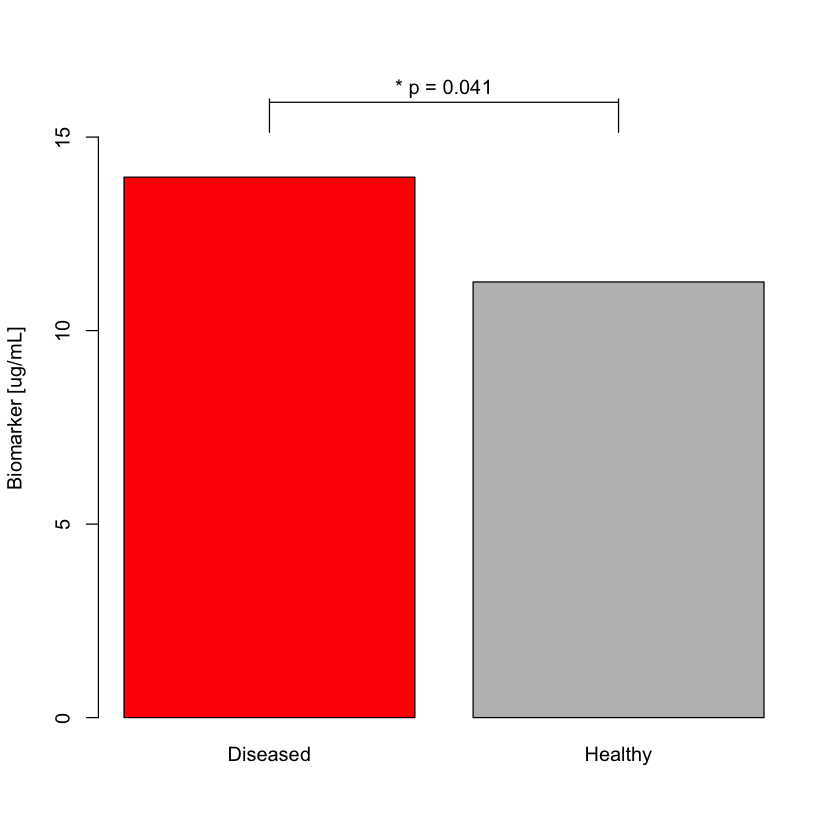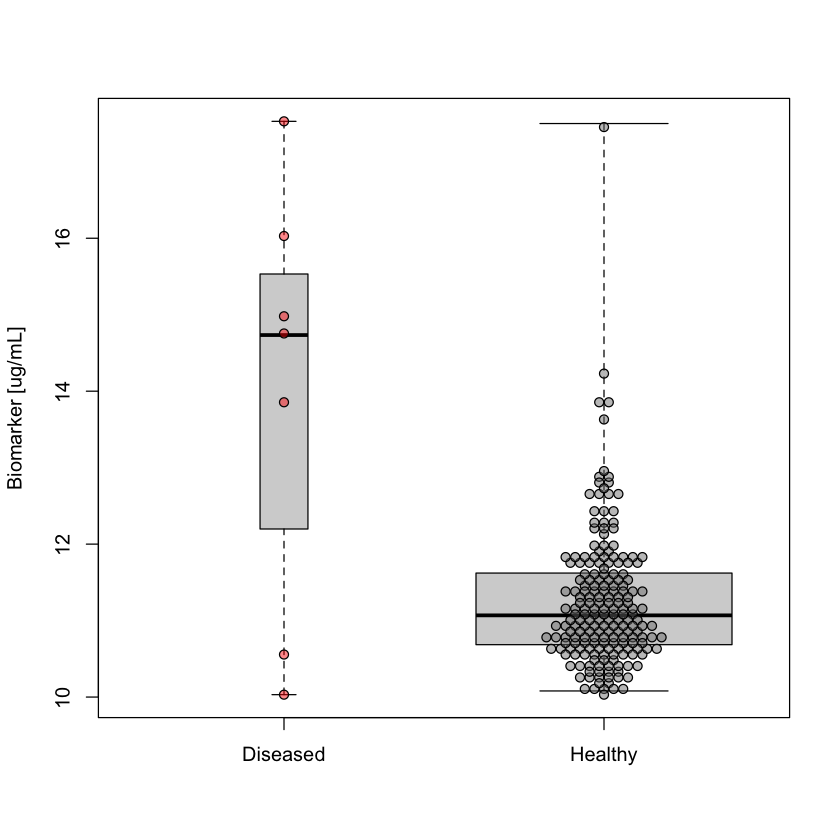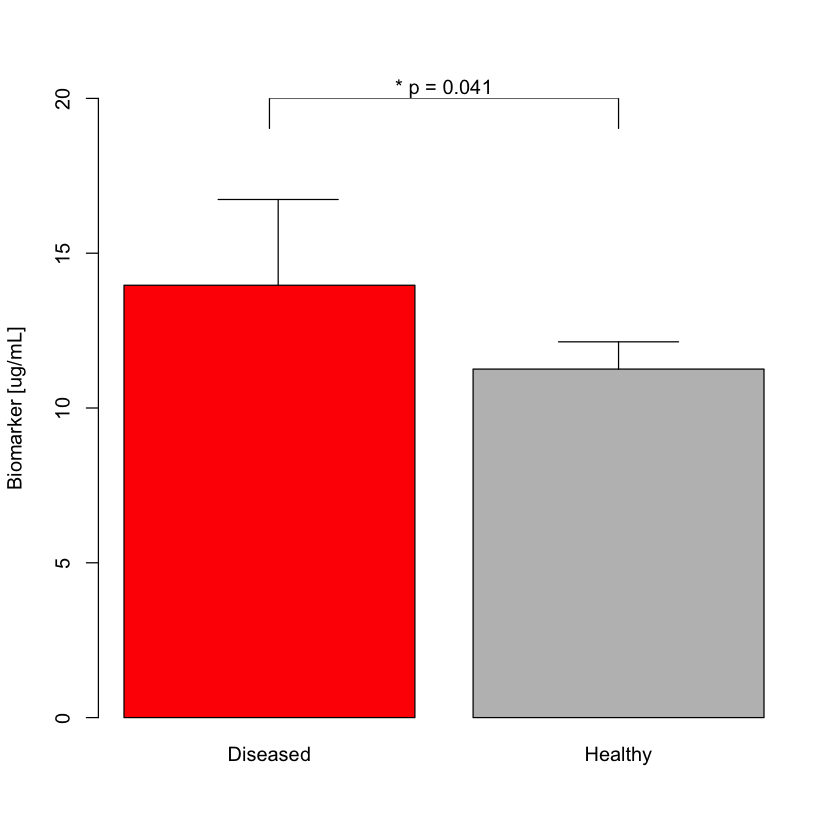Select Page

There are multiple ways to present the data, some are better than others. However, it seems that researchers, especially in the field of life sciences, are particularly fond of one form of data presentation: the barplot.

## The bar plot – how good is it?¶

Consider the following example. Let’s say we measured a biomarker level in two groups of patients: those with and without the disease. Many would agree that it is very convenient to present the mean biomarker levels in each group in the form of bars, as the difference in height of the bars is very easily judged by the human eye, and therefore the plot conveys the point in a simple form. We can even add a p-value for comparing the groups to the plot, to make it look more “scientific” and less “business-like”. See the following example:

In :
# Random generation of "biomarker" values for two groups
set.seed(102)
diseased = c(rbeta(5,10,2), rbeta(2,2,10))*10+8
healthy = c(rgamma(199, 2.5,20)*10+10,17.5)

# The barplot
barplot(c(Diseased=mean(diseased), Healthy=mean(healthy)), col=c("red","gray"), ylab="Biomarker [ug/mL]", ylim=c(0,16))
arrows(0.7,15.9,1.9,15.9, angle=90, code=3)
mtext(paste("* p =",round(t.test(healthy, diseased)$p.val,3)), side=3, adj=0.5)Let’s summarize the advantages and disadvantages of this kind of plot. The advantages are: it is simple and conveys the point. The disadvantages are: where do I start… Let me just say this: back in the olden days, when I was young and foolish, I would take such plot at face value. Nowadays, when I see such a plot, the first thing that comes to my mind is a question: “what are they trying to hide?” Let’s review the actual data behind this plot: In : # Combine the measurements into a data set a = data.frame(Status=c(rep("Healthy",200), rep("Diseased",7)), Biomarker=c(healthy, diseased)) a$Status = factor(a$Status) # Generate box & whisker plot boxplot(Biomarker~Status, data=a, range = 0, varwidth = T, xlab="", ylab="Biomarker [ug/mL]") # Overlay a dot-plot on top of it par(new = T) accu = diff(range(a$Biomarker))/100
stp  = 0.03
x    = as.integer(a$Status) y = round(a$Biomarker/accu) * accu
x1   = unique(x)
for (i in seq(along = x1)) {
freq = table(y[x == x1[i]])
for (j in seq(along = freq)) {
if (freq[j] >= 2) {
offset = (freq[j] - 1)/2 *  stp
for (k in seq(along = y)) {
if (abs(y[k] - as.numeric(names(freq)[j])) < 1e-10 && abs(x[k] - x1[i]) < 1e-10) {
freq[j] <- freq[j] - 1
x[k] <- x[k] - offset + freq[j] * stp
}
}
}
}
}
plot(x, y, axes=0, xlim=c(0.5,2.5), xlab="", ylab="", pch=21, bg=c("#ff000077","#77777777")[a$Status])A-ha! It is now clear that there are only 7 measurements in the “Diseased” group (perhaps it’s a rare disease). Secondly, the range of measurements is the same for “Diseased” and “Healthy” groups. In the “Healthy” group most of the results are bunched at the bottom of the plot, while in the “Diseased” group measurements are spread more or less evenly across the scale. That is how the real data look like. Now take a look back at the barplot. Do you see the real data in there? The fact is: barplot makes it very hard (if not impossible) for the reader to assess the validity of results and quality of the claim people make in their study. On the other hand, for the researcher barplot makes it easy to hide flaws of the study, such as imbalanced groups or high spread of measurements. Can the barplot be fixed? ## If your data is behind bars – blow them up!¶ Yes, blow up the bars of your data’s prison and set it free! The best way to do this is obviously using some explosives and a detonator. Joking aside, clever people found a way to make the barplot appear more appropriate by adding a whisker that represents the Standard Deviation of the underlying data on top of the bar. In : barplot(c(Diseased=mean(diseased), Healthy=mean(healthy)), col=c("red","gray"), ylab="Biomarker [ug/mL]", ylim=c(0,20)) arrows(0.73, mean(diseased), 0.73, mean(diseased)+sd(diseased), 0.5, angle=90, code=2) arrows(1.9, mean(healthy), 1.9, mean(healthy)+sd(healthy), 0.5, angle=90, code=2) arrows(0.7,20,1.9,20, angle=90, code=3) mtext(paste("* p =",round(t.test(healthy, diseased)$p.val,3)), side=3, adj=0.5)And hence the dynamite plot was born. It just begs to press down on that lever…

## How to do this right?¶

Use a box & whisker plot, or better yet – a so-called dot-plot. Or better yet – a combination of box & whisker plot with the so-called dot-plot, as shown in the code snippet  above.

That kind of plot is insensitive to underlying distribution: it can be skewed, bi-modal, tri-modal, whatever – it all shows through. This kind of plot exposes all the data to the viewer’s eye and allows them to make their own judgement. And at the same time it provides basic points of reference: where the median and quartiles are located. Perfect!

Then why so many people don’t want it?

Because if I saw a plot like the one in  with results like the ones in  – I would move on to the next poster…

## Bars do have their place in science¶

It wouldn’t be honest on my part to leave this matter as it stands above. There is a rightful place for barplot in scientific data presentation. This kind of plot is perfectly valid for displaying count data, such as number of enrolled patients, number of performed procedures, etc. It is also very useful in business setting – for example for comparing prices or revenues. Lastly, I’ve seen it used cleverly to display proportions of count items, such as carriers of a specific mutation within patient groups, etc.